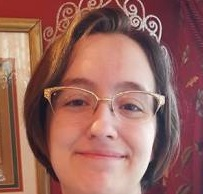# 7.2: Verb Tenses: Active Voice

•• Contributed by Robing Jeffrey
• Learning Resource Center (LRC) Director at Klamath Community College

## SIMPLE TENSES

### SIMPLE PRESENT

$$\text{General facts, states of being, scheduled events in the future, and repeated actions}\; = \text{base form or –s form}$$

Example $$\PageIndex{1}$$:

• Teachers often grade late into the night.
• Water becomes ice at 32 degrees Fahrenheit.
• Celebrities donate to hundreds of charities every year.
• The plane departs tonight at 9:00 p.m.

### SIMPLE PAST

$$\text{Completed actions from the past that occurred at a specific time or facts/states of being that occurred in the past} = \text{base form} + \text{-ed/-d/irregular form}$$

Example $$\PageIndex{2}$$:

• Their neighbors worked together to rebuild the house.
• He drove across country to prove a point.
• When she was young, my sister played with me all the time.

### SIMPLE FUTURE

$$\text{Future actions, predictions, or promises} = \text{will} + \text{base form}$$

Example $$\PageIndex{3}$$:

• I will eat in a few minutes.
• The rain will stop any second now.

## SIMPLE PROGRESSIVE FORMS

### PRESENT PROGRESSIVE

$$\text{Actions that are happening right now, but not happening forever or future actions} = \text{am, is, are} + \text{present participle}$$

Example $$\PageIndex{4}$$:

• The teachers are meeting in the boardroom. Josie is meeting the principal.
• We are baking cookies tomorrow.

### PAST PROGRESSIVE

$$\text{Actions that happened at a specific time in the past or past plans that didn’t happen} = \text{was, were/was going to, were going to} + \text{present participle}$$

Example $$\PageIndex{5}$$:

• They were sailing when the hurricane hit.
• I was going to fly out tonight, but couldn’t get a ticket.

## PERFECT TENSES

### PRESENT PERFECT

$$\text{Repetitive or constant actions that began in the past but are still happening or actions that happened at an unspecified time in the past} = \text{has, have} + \text{past participle}$$

Example $$\PageIndex{6}$$:

• I have loved dogs since I was a child.
• Alex has lived in the UK for over a year.
• Stephanie has bought three cars in three years.

### PAST PERFECT

$$\text{Actions that occurred/began before something else in the past} = \text{had} + \text{past participle}$$

Example $$\PageIndex{7}$$:

He had just choked when the ambulance drove by.

## PERFECT PROGRESSIVE FORMS

### PRESENT PERFECT PROGRESSIVE

$$\text{Continuous actions that began in the past but are still occurring} = \text{has, have} + \text{been} + \text{past participle}$$

Example $$\PageIndex{8}$$:

Ygritte has been trying to learn knitting for years.

### PAST PERFECT PROGRESSIVE

$$\text{Actions that began and continued in the past until some other action in the past occurred} = \text{had} + \text{been} + \text{present participle}$$

Example $$\PageIndex{9}$$:

By the time I moved to Klamath Falls, I had been writing for ten years.